# A.6 Spatial inertia

Assume we have a rigid body with mass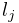and a coordinate frame located at the body’s center of mass. If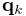and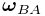give the translational and rotational velocity of the coordinate frame, then the body’s linear and angular momentum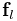and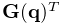are given by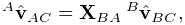(A.30)

where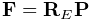is the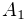rotational inertia with respect to the center of mass. These relationships can be combined into a single equation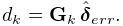(A.31)

where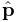and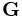are the spatial momentum and spatial inertia: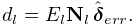(A.32)

The spatial momentum satisfies Newton’s second law, so that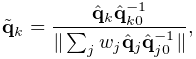(A.33)

which can be used to find the acceleration of a body in response to a spatial force.

When the body coordinate frame is not located at the center of mass, then the spatial inertia assumes the more complicated form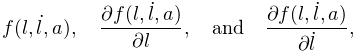(A.34)

where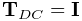is the center of mass and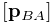is defined by (A.22).

Like the rotational inertia, the spatial inertia is always symmetric positive definite if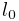.